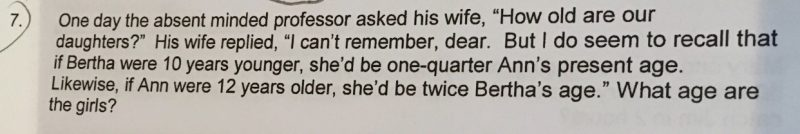# QuestionCan anyone help?  Not sure whether is my methodology is accurate or wrong.

10 years younger

Bertha: 1/4x-10

Ann: x

12 years older

Bertha: 1/2x

Let Bertha’s age be x and Ann’s age be y.

Equation (1): x – 10 = 0.25y

Equation (2): y + 12 = 2x

If we rearrange (2) , we get y = 2x – 12

We then sub (2) into (1), x – 10 = 0.25(2x – 12).

We get x = 14, y = 16

0 Replies 1 Like ✔Accepted Answer

B-10 = 1/4A
A+12 = 2 B

(B-10)x 4 + 12 = 2 B

4B – 40 +12 = 2 B
4B-2B = 40-12
2B = 28
B = 14
A = (14-10)x4 = 16

in my opinion

0 Replies 1 Like